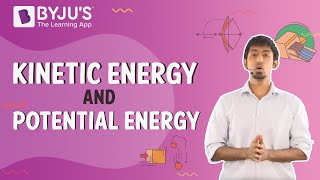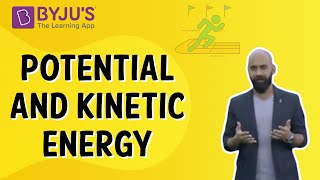# Energy Questions

## What is Energy

The ability to do work is termed energy; there are diverse forms of energy on this planet. Energy is considered a measurable property in physics, which can be transferred from one object to another to accomplish or perform work.

The law of energy conservation states that “The energy can only be transformed from one form to another. It can neither be created nor be destroyed”. Joule is the SI unit of energy.

### Units of Energy

According to the International System of Units, energy is measured in joules. Energy is also measured in many other units which are not part of the SI, such as calories, ergs, etc.

Energy can be transferred from one object to another in the following ways:

• Through Heating – Energy can be transferred in heat using three processes: conduction, convection, and radiation.
• Mechanically – Energy can be transferred mechanically using the action of force.
• Electrically – Using electric power and circuits, energy can be transmitted electrically.
• Radiation – The phenomenon of radiation is used by light waves or sound waves to transmit energy.

### Law of Conservation of Energy

One of the most fundamental laws in physics is the law of conservation of energy. The law of conservation of energy states that the system’s total energy is conserved in a closed system, that is, a system that is isolated from its surroundings”.

## Important Questions on Energy

1) What is kinetic energy?

The form of energy that an object or a particle possesses by virtue of its motion is termed as kinetic energy.

The kinetic energy of a body is equal to the product of the square of its velocity, v and one-half the product of its mass, m, that is, K.E. = 1/2mv2.

2) What are the different types of potential energy?

There are basically four types of potential energy:

1. Gravitational potential energy

2. Elastic potential energy

3. Chemical potential energy

4. Electric potential energy

3) What kind of energy does a flying bird possess?

a) Kinetic energy

b) Potential energy

c) Chemical energy

d) Both potential and kinetic energy

Correct Option: (d)

Explanation: A flying bird possesses both potential as well as kinetic energy. At a certain height of the flight, the bird maintains a certain kind of constant velocity. This means that the changing height is responsible for potential energy, and the horizontal motion with a certain kind of velocity is responsible for kinetic energy.

4) What happens to the potential energy of the spring when it is stretched or compressed?

a) Increases

b) Remains constant

c) Decreases

d) Becomes zero

Correct Option: (a)

Explanation: When a spring is compressed or stretched, then due to the work done on the spring; it’s the potential energy increases.

5) What will happen to the kinetic energy of a body when its momentum is doubled?

a) Kinetic energy remains the same

b) Kinetic energy will drop to zero

c) Kinetic energy will become four times its original value

d) Kinetic energy will become ten times its original value

Correct Option: (c)

Explanation: Since, K.E. = 1/2 mv2 = (mv)2/2 m = p2/2 m

K α p2

Therefore, when the K.E. is proportional to the square of the momentum of a body, K.E. becomes four times its original value.

6) What is the correct sequence of energy change from one form to another in a thermal power station when coal is used for generating electricity?

a) Heat energy > Chemical energy >Electrical energy

b) Mechanical energy >Electrical energy > Heat energy

c) Heat energy >Mechanical energy >Electrical energy

d) Chemical energy >Heat energy >Mechanical energy

Correct Option: (c)

Explanation: The combustion of coal converts water into steam when heat energy is produced by it. This heat energy of steam turns the blades of a turbine, which means that it is converted into mechanical energy. Then finally, the mechanical energy attained is transformed into electrical energy by the generators.

7) What will happen to the momentum of an aeroplane when its velocity is doubled?

a) Remain unchanged

b) Momentum will be doubled

c) Becomes zero

d) Momentum increases uniformly

Correct Option: (b)

Explanation: Since, p = mv is the formula for momentum, where m = mass, v = velocity and p = momentum. Therefore, if the velocity of the aeroplane is doubled, its momentum is also doubled.

8) The temperature of water at the top of the fall will be different from the temperature at the foot of the fall.

a) True

b) False

Correct Option: (a)

Explanation: The velocity of water is maximum at the foot of the fall because potential energy is converted into kinetic energy when the water falls from a height. At the bottom of the fall, the velocity is rapidly reduced to zero, and hence Its kinetic energy gets transformed into heat energy at the bottom, which raises its temperature.

9) Define the law of conservation of energy.

One of the most basic laws in the world of physics is the law of conservation of energy. The microscopic motion of individual atoms is ruled by this law in a chemical reaction. The law of conservation of energy states that “The energy can only be transformed from one form to another, it can neither be created nor be destroyed”. According to this law, “The total energy of the system is conserved in a closed system, that is, a system that is isolated from its surroundings”.

10) When one proton and one electron are brought close together, what will happen to the potential energy?

a) P.E. increases

b) P.E. gets converted into K.E.

c) P.E. remains the same

d) P.E. decreases

Correct Option: (d)

Explanation: Because the work is done by the force of attraction between the electron and proton, the potential energy decreases when they are brought closer to each other.

### Practice Questions

1) Differentiate between kinetic energy and potential energy.

2) Define energy and its types.

3) What is thermal energy?

4) What is gravitational potential energy?

5) What is the commercial unit of energy?

 Related Articles

## Suggested VideosStay tuned to BYJU’S and Fall in Love with Learning!